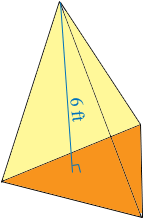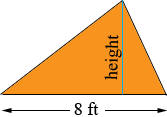SEARCH HOMEMath Central Quandaries & QueriesQuestion from joe, a parent: triangular pyramid base height= base width=8ft height=6ft volume=88ftcu. Please explain base height & how to solve.Joe,

The main fact you need is that the volume of a triangular pyramid is one-third the area of the base, times the height. In your pyramid the height is 6 feet.The base of the pyramid is a triangle and the area of a triangle is one-half the base times the height.What must the height of the base be if the volume of the pyramid is 88 cubic feet?

PennyMath Central is supported by the University of Regina and The Pacific Institute for the Mathematical Sciences.